Worksheets and No Prep Teaching Resources
Math Worksheets
Place Value Worksheets

# Billions and TrillionsWorksheets, Lessons, and Printables

Place Value No Prep Book #1
Place Value No Prep Book #1 - Not Boring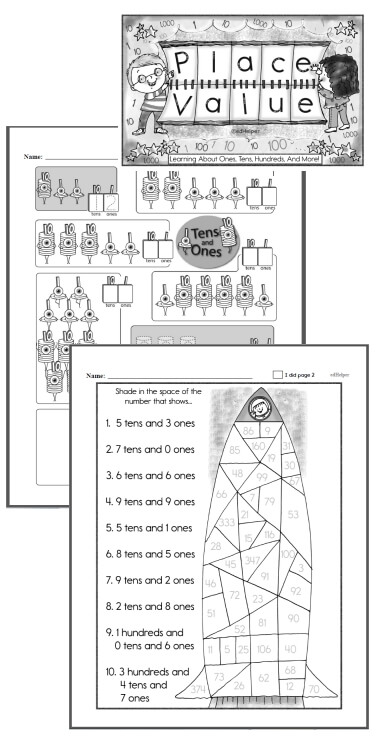Kindergarten - No Prep Place Value Book First Grade - No Prep Place Value Book Second Grade - No Prep Place Value Book

Large Numbers: billions and trillions
Large Numbers billions and trillions - Write the Place Values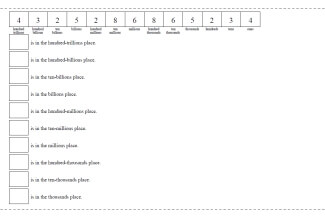Fill in all the places (text hints given for all problems) Fill in all the places (text hints given for first problem) Fill in all the places (no text hints)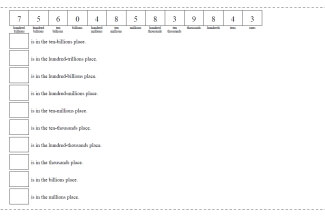Fill in all the places - random order (text hints given for first problem) Fill in all the places - random order (no text hints)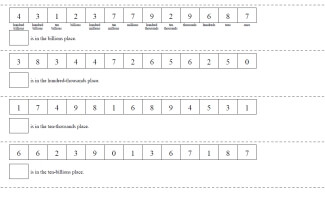Fill in one place (text hints given for first problem) Fill in one place (no text hints)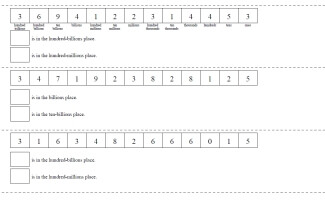Fill in two places (text hints given for first problem) Fill in two places (no text hints)

Large Numbers billions and trillions - Write the Number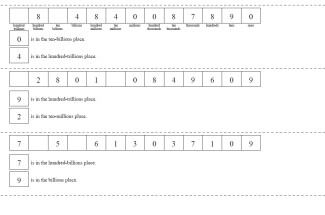Complete the Number: two places given (text hints given for first problem) Complete the Number: two places given (no text hints)

Large Numbers billions and trillions - Making a Number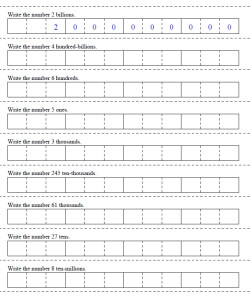Make a number from one place value (first answer given) Make a number from one place value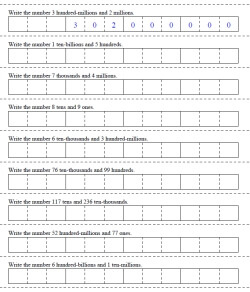Make a number by adding two place values (first answer given) Make a number by adding two place values

Place Value

Have a suggestion or would like to leave feedback?